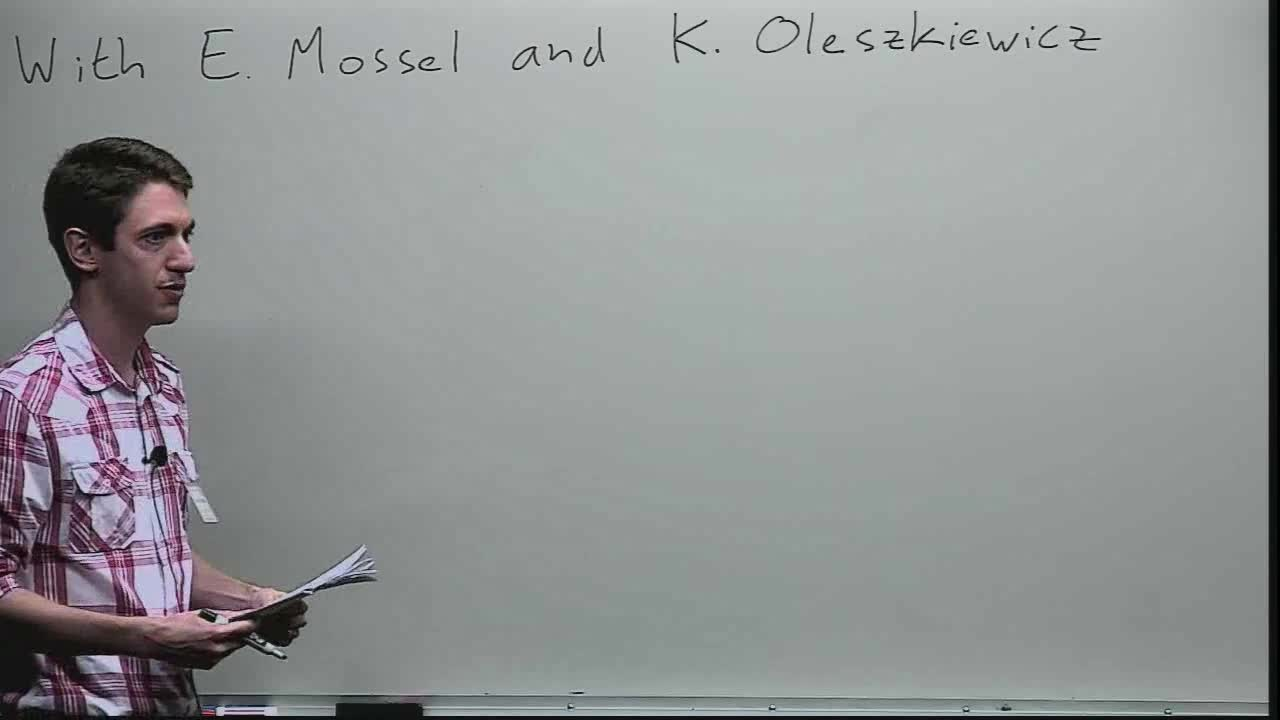## Strong Contraction and Influences in Tail Spaces.

April 28, 2015
IMA
Presenters: Steven Heilman

Watch Video

#### Abstract

We study contraction under a Markov semi-group and influence bounds for functions all of whose low level Fourier coefficients vanish. This study is motivated by the explicit construction of 3-regular expander graphs of Mendel and Naor, though our results have no direct implication for the construction of expander graphs. In the positive direction we prove an Lp Poincaré inequality and moment decay estimates for mean 0 functions and for all finite p>1, proving the degree one case of a conjecture of Mendel and Naor as well as the general degree case of the conjecture when restricted to Boolean functions. In the negative direction, we answer negatively two questions of Hatami and Kalai concerning extensions of the Kahn-Kalai-Linial and Harper Theorems to tail spaces. For example, we construct a function f:{-1,1}^n -> {-1,1} whose Fourier coefficients vanish up to level c log n, with all influences bounded by C(log n)/n for some constants c,C>0. That is, the Kahn-Kalai-Linial Theorem cannot be improved, even if we assume that the first c log n Fourier coefficients of the function vanish. This implies there is a phase transition in the largest guaranteed influence of functions f:{-1,1}^n -> {-1,1}, which occurs when the first g(n)log n Fourier coefficients vanish and g(n) increases to infinity as n goes to infinity, or g(n) is bounded as n goes to infinity. Joint with Elchanan Mossel and Krzysztof Oleszkiewicz.